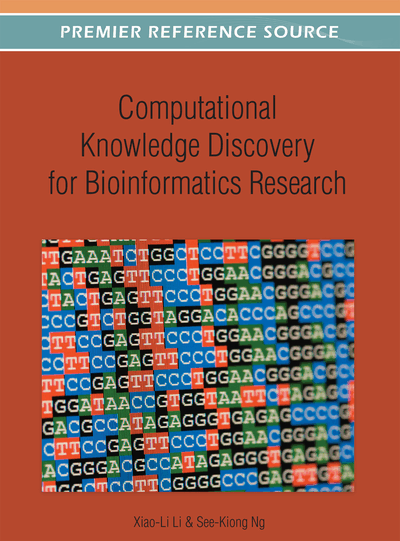# Identification of Distinguishing Motifs

Wangsen Feng (Peking University, China) and Lusheng Wang (City University of Hong Kong, China)
DOI: 10.4018/978-1-4666-1785-8.ch001
Available
\$33.75
List Price: \$37.50
10% Discount:-\$3.75
TOTAL SAVINGS: \$3.75

## Abstract

Motif identification for DNA sequences has many important applications in biological studies, including diagnostic probe design, locating binding sites and regulatory signals, and potential drug target identification. There are two versions—the Single Group and Two Groups. Here, the occurrences of the motif in the given sequences have errors. Currently, most of existing programs can only handle the case of single group. However, most of the programs do not allow indels (insertions and deletions) in the occurrences of the motif. In this paper, the authors propose a randomized algorithm for the one group problem that can handle indels in the occurrences of the motif. Finally, an algorithm for the two groups’ problem is given along with extensive simulations evaluating algorithms.
Chapter Preview
Top

## 1. Introduction

Motif identification for DNA sequences has many important applications in biological studies, e.g., diagnostic probe design, locating binding sites and regulatory signals, and potential drug target identification (Dopazo, Rodriguez, Saiz, & Sobrino, 1993; Lucas, Busch, Mossinger, & Thompson, 1991; Proutski & Holme, 1996). The most general problem is as follows:

• Two Groups: Given two groups of sequences B and G, find a length-l (distinguishing) motif that appears in every sequence in B and does not appear in anywhere of the sequences in G.

The motif we want to find is called the distinguishing motif that can differentiate the two groups. If no error is allowed, the problem is easy. However, in practice, the occurrence of the motif in each of the given sequences has errors (mutations and indels). The problem becomes extremely hard when errors appear. Many mathematic models have been proposed.

The following definition was first stated in (Lanctot, Li, Ma, Wang, & Zhang, 1999).

• Distinguishing substring selection: given a set B = {s1, s2,…, sn1} of n1 (bad) strings of length at least l, and a set G = { g1,g2, …, gn2} of n2 (good) strings of length at least l, the problem is to find a string s of length l such that for each string si∈B there exists a length-l substring ti of si with dH(s, ti)≤ db and for any length-l substring ui of gi∈ G, dH(s, ui)≥ dg. Here db and dg are two parameters, called the radius of the two groups. We want db to be small and dg to be large. dH(,) represents the Hamming distance between two strings.

When indels are allowed in the occurrences of the motif, the problem is called the distinguishing subsequence selection problem, where the input is the same as the previous problem and the objective here is to find a string s of length l such that for each string si ∈ B there exists a substring ti of si with d(s, ti) ≤ db and for any substring ui of gi ∈ G, d(s, ui) ≥ dg. Here d(,) represents the edit distance.

A special case, where only one group of sequences is involved is as follows:

• Single Group: Given a group of n given sequences, find a motif that appears in each of the given sequences and those occurrences of the motif are similar.

There are several ways to define the similarity of the motif occurrences, e.g., the consensus score, general consensus score and SP-score (Hertz & Stormo, 1995; Li, Ma, & Wang, 1999). Here we use the following mathematical definitions.

is minimized, where dH (,) is the Hamming distance between the two strings of the same length.

## Complete Chapter List

Search this Book:
Reset